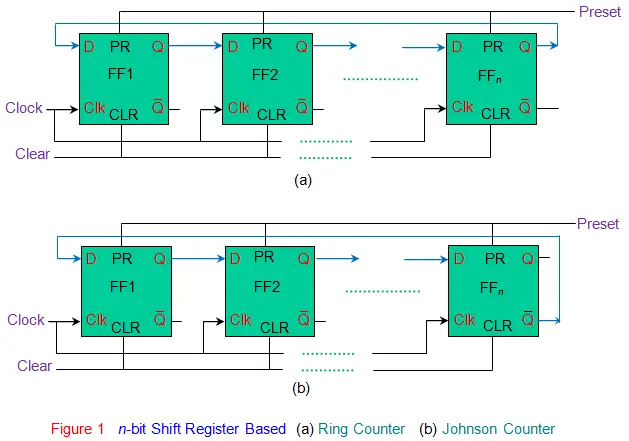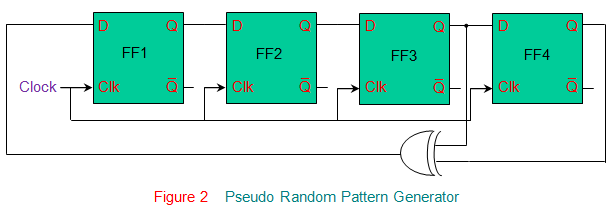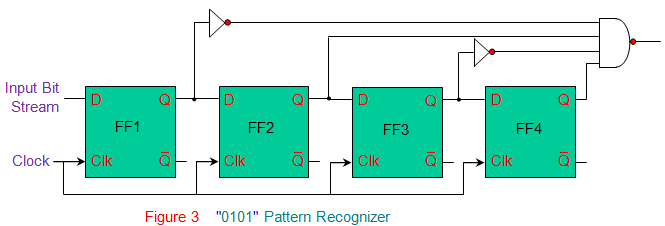# Applications of Shift Registers

### Delay Lines of Shift Registers

Shift registers of Serial In Serial Out (SISO) kind can be used to delay the digital signals by a definite period time. The time delay introduced by the n-bit shift register is equal to n times the inverse of the clock frequency driving the shift register. Very long shift registers of this kind can be used as delay line memory in computer systems to store the temporary data. Further, multiple bidirectional shift registers connected in parallel can be used as a stack.

### Data Format Converters of Shift Registers

Serial data transmission is preferred for long distance communication due to its economical value in terms of the wires used. This necessitates parallel-to-serial conversion at the sender-end for which Parallel In Serial Out shift registers (PISO) can be used. However, in general, many microprocessor based systems usually prefer parallel form of data-in for which the transmitted data is to be converted into parallel-mode using serial-to-parallel converter like Serial In Parallel Out shift register (SIPO).

### Data Processors of Shift Registers

Earlier computer systems used shift registers to process the data. For example, shift-registers were used to store the data bytes which were to be added as well as to store their result. Moreover even now the shift registers are extensively used to perform binary multiplication and division. This is due to the fact that the shift of data bit by one position towards right causes the number to be divided by 2 while the left-shift of the data bit by one place in the shift register multiplies the number by 2. For example, consider a 4-bit shift register with the content 0110, which is equal to 6 in decimal. If the number shifts left by one-bit, then one gets 1100, which is 12 (= 6 × 2) in decimal. Similarly is the number shifts towards right by one bit, then the register contents will become 0011, which is nothing but 3 (= 6/2) in decimal.

### Counters of Shift Registers

Ring Counter and the Johnson Counter are the two shift-register based counters which are extensively used in digital applications. In ring counters the output of the last stage is back-fed as an input to the first stage as shown by Figure 1a. This causes the data stored within the shift register to circulate within it continuously. For example, a 4-bit ring counter storing a data word 0001 has repetitive sequence with four definite states viz., 0001, 1000, 0100 and 0010. Johnson Counter shown by Figure 1b is similar to ring counter except the fact that the complement of the output at the last stage of the shift-register is fed as an input to the first stage.

Thus for a 4-bit Johnson counter with input data word as 0001, one gets eight definite states – 0001, 0000, 1000, 1100, 1110, 1111, 0111 and 0011 – after which the pattern repeats.### Pseudo Random Pattern Generator of Shift Registers

Shift registers can be used to generate pseudo random patterns which are used for testing. In order to achieve this, the outputs of few stages in the shift register are EX-ORed and connected as an input to the first stage of it, as shown by Figure 2.The number of patterns generated depend upon the number of points which are tapped to be provided as X-OR gate inputs. If tapped appropriately, the maximum number of patterns that can be generated using a n-stage shift register is (2n-1).

### Pulse Extenders of Shift Registers

Pulse Extenders are the circuits which have longer output pulses when compared to the input pulses. Shift registers can be used as pulse extenders and are advantageous over mono stable multi vibrators as the timing is independent of the component values. However they are operated by an external clock which in turn determines the timing accuracy.

### Pattern Recognizers of Shift Registers

Shift registers can be used to determine the occurrence of a definite bit pattern at the input. For example, the Figure 3 shows a 0101 pattern recognizer formed by using shift registers and the NAND gate. Here, as the sequence of bits in the input stream becomes 0101, the output of the NAND gate goes low.### Interconnectors of Shift Registers

Shift registers of the type SISO and PISO can be effectively used to reduce the number of wires interconnecting the various systems in the design. For example, in order to drive 16 LEDs from a microcontroller one would require 16 General Purpose Input Output (GPIO) ports. Instead two shift registers connected in series can be used to complete the task just by using 4 Input/Output (I/O) pins.

Want To Learn Faster? 🎓
Get electrical articles delivered to your inbox every week.
No credit card required—it’s 100% free.